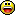# Painting

Posted By: Emre

## Painting - 09/26/19 09:07

Hi guys, i'm trying to paint texture/bmap.

this is the code i use:

Code
```
#include <acknex.h>
#include <windows.h>
#include <default.c>

BMAP* paint_map="#512x512x24";

PANEL* paint_pan =
{
bmap=paint_map;
flags=SHOW;
}

var brush_size=32;

function main()
{
fps_max=75;
video_set(512,512,32,2);

mouse_mode=3;
mouse_pointer=2;
bmap_fill(paint_map,vector(255,255,255),100);

var pic_width=bmap_width(paint_map);
var pic_height=bmap_height(paint_map);
var pixel;
var format;
var px,py;

while(1)
{

if(mouse_left)
{
format = bmap_lock(paint_map, 888);

int i=0;
for(i=0;i<brush_size; i++)
{
int j=0;

for(j=0;j<360;j++)//circle
{

pixel = pixel_for_vec(vector(0,255,0),100,format);
px=clamp(mouse_pos.x+i*cos(j),1,pic_width-1);
py=clamp(mouse_pos.y+i*sin(j),1,pic_height-1);

pixel_to_bmap(paint_map, px,py, pixel);

}

}
}
bmap_unlock(paint_map);

wait(1);
}

}

```

i need soft brush and pressure option, as in image editing programs.. Any help would be great.Posted By: txesmi

## Re: Painting - 09/26/19 22:01

Hi,
here you go
Code
```void col_lerp(COLOR *_cT, COLOR *_c0, COLOR *_c1, var _factor, var _gamma) {
_cT->red   = pow(pow(_c0->red, _gamma) * (1 - _factor)   + pow(_c1->red, _gamma) * _factor,   1.0 / _gamma);
_cT->green = pow(pow(_c0->green, _gamma) * (1 - _factor) + pow(_c1->green, _gamma) * _factor, 1.0 / _gamma);
_cT->blue  = pow(pow(_c0->blue, _gamma) * (1 - _factor)  + pow(_c1->blue, _gamma) * _factor,  1.0 / _gamma);
}

void bmap_paint_circle (
BMAP *_bmp,
int _posX,
int _posY,
COLOR *_color,
var _alpha)
{
if (_g == 0)
_g = 0.001;
int _xL = minv(_radiusExt, bmap_width(_bmp) - _posX);
int _yL = minv(_radiusExt, bmap_height(_bmp) - _posY);
var _frt = bmap_lock(_bmp, 0);
for (; _y<_yL; _y+=1) {
int _x = _x0;
for (; _x<_xL; _x+=1) {
var _r = sqrt(_y * _y + _x * _x);
continue;
var _f = (1 - maxv(_r - _radiusInt, 0) / _g) * _alpha / 100;
var _p = pixel_for_bmap(_bmp, _posX + _x, _posY + _y);
COLOR _c;
pixel_to_vec(&_c, NULL, _frt, _p);
col_lerp(&_c, &_c, _color, _f, 2.2);
//				vec_lerp(&_c, &_c, _color, _f);
_p = pixel_for_vec(&_c, 100, _frt);
pixel_to_bmap(_bmp, _posX + _x, _posY + _y, _p);
}
}
bmap_unlock(_bmp);
}
```

Salud!
Posted By: Emre

## Re: Painting - 09/27/19 03:45

This is awesome! Thank you very much, txesmi!
Posted By: txesmi

## Re: Painting - 09/27/19 14:49

You are welcome